From 3A TO 4A

51 10 55.57 N 1 47 15.76 W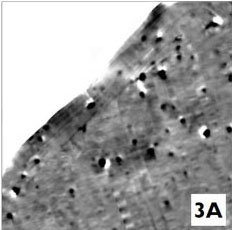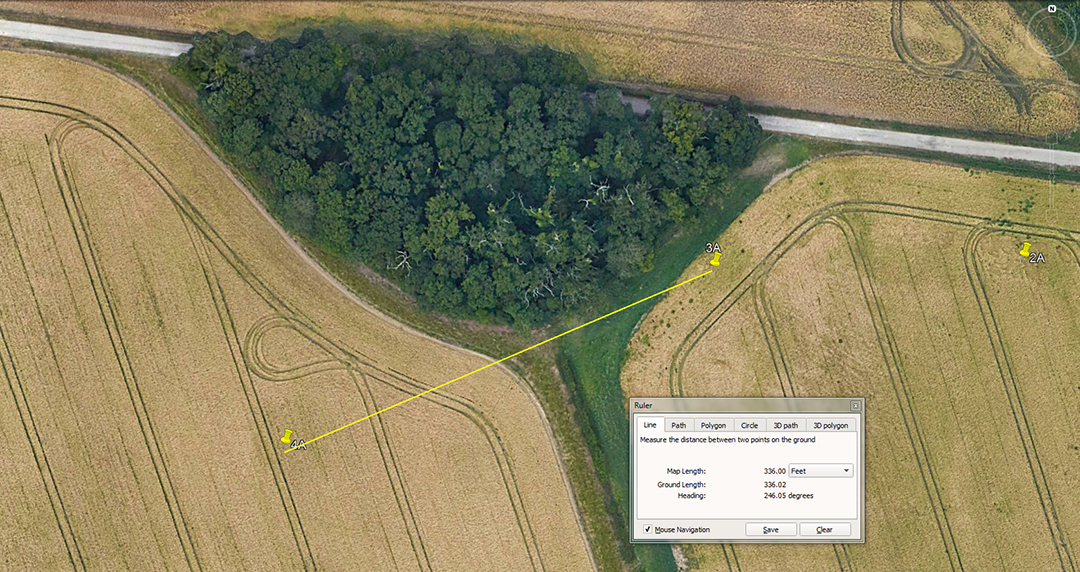The distance is 336-feet and the angle is 246-degrees (66-degrees return).

A mathematical progression based upon 336 goes:

336, 672, 1008, 1344, 1680, 2016, 2352, 2688, 3024, 3360, 3696, 4032, 4368, 4704, 5040, 5376, 5712, 6048 ... 6720 ... 9072 ... 25200, etc.

In the above lineup the sum of 100.8-feet would be 1-second of equatorial arc, 3024-feet would be the distance covered for 1 circumnavigation of the Great Pyramid and half a minute of arc, 6048 would be 1-minute of arc, 9072-inches would be the length of 1 side of the Great Pyramid, 25.2-inches would be 2 Greek feet of 12.6-inches each or 1 Assyrian cubit, etc.

All of the above will be perfect whole number fractions of the equatorial size of the Earth for the Great Pyramid's literal navigational system, rendered in feet. Thus, 130636800-feet (24883.2 Greek miles of 5250-feet) ÷ 6048 = 43200.

The degree angle is in homage to the 5280-feet mile system of navigation (the "11" number family, used in ancient England and Scotland as elsewhere, with the ancient Scottish mile being 5940-feet).

The progression goes:

66, 132, 198, 264, 330, 396, 462, 528, 594, 660, 726, 792, 858, 924, 990, 1056, etc. The progression advances to 5280, 5940, 7920, 16500, and ultimately to 130680000-feet for the equatorial circumference of the Earth under the English mile of 5280' or Scottish mile of 5940'.

In the above line-up of numbers, 66-feet would be an ancient English chain, 660-feet would be a furlong or furrowlong, 5280-feet an English mile, 5940-feet a Scottish mile, 16500-feet an ancient English league (3.125-miles of 5280-feet), 16.5-feet would be a rod or perch (3 merchant navy fathoms of 5.5-feet ... still in use in the early 20th century) and 7920-miles would be the diameter of the Earth.

The merits of 337.5-feet would also have been taught, as well as 340.2'.

A mathematical progression based on 337.5 goes:

337.5, 675, 1012.5, 1350, 1687.5, 2025, 2362.5, 2700, 3037.5, 3375 ... 4050 ... 4725 ... 5062.5, 5400 ... 6075 ... 7087.5 ... 11812.5, etc.

The string relates mainly to lunar coding and there would be 3.5 X 101.25-days in a lunar year of 354.375-days. In the 2835-feet circuit around the Khafre Pyramid of Egypt (a pyramid of the moon ... 708.75-feet per side) there would be 28 increments of 101.25-feet. The sum of 118.125-days = 4 lunar months of 29.53125-days (29 & 17/32nds) ... accurate to a tolerance of less than a minute in time per lunar month.

The Khafre Pyramid was also built as 2 back-to-back 3-4-5 triangles founded upon lunar coding. Half the base length was 354.375-feet (118.125 X 3), The design, vertical height was 472.5 feet (118.125 X 4) and the diagonal slope, side length was 590.625-feet (118.125 X 5).

4A TO 5A

51 10 54.26 N 1 47 20.59 W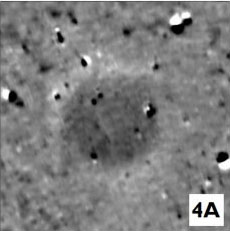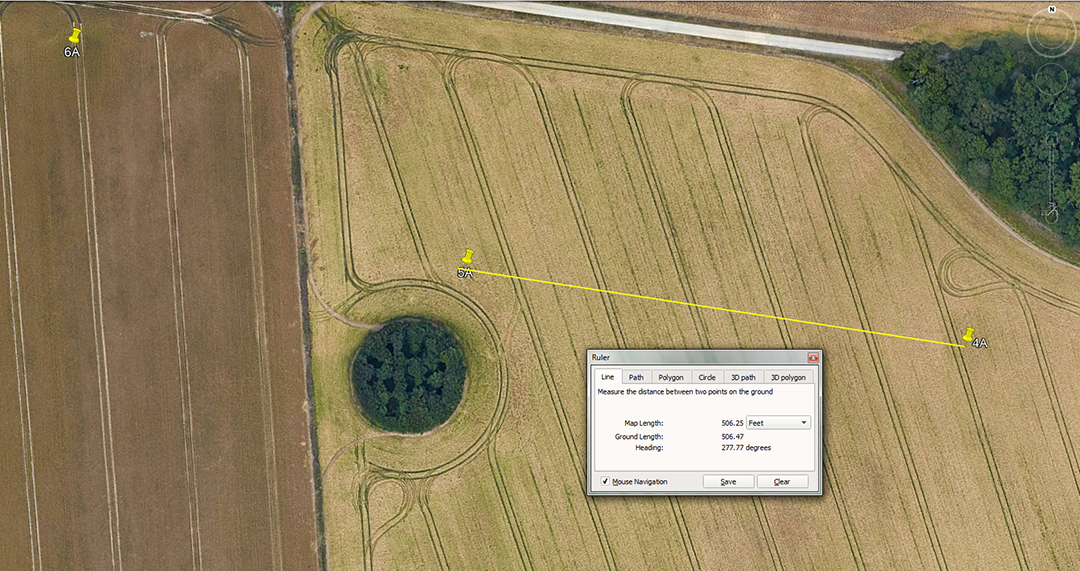The distance is 506.25-feet and the angle is 277.7777-degrees. The merits of 280-degrees would also have featured in the tutorial.

A mathematical progression based upon 506.25 goes:

506.25, 1012.5, 1518.75, 2025, 2531.25, 3037.5, 3543.75 ... 7087.5, etc.

This is, of course, lunar coding.

A mathematical progression based upon 277.77777 (277 & 7/9ths) goes:

277.77777, 555.55555, 833.33333, 1111.11111, 1388.88888, 1666.66666, 1944.44444, 2222.22222, 2500.

The ancient mathematicians were using all of the number families for their calculations, including dividing up in 7ths. Thus a much favoured form of PI, essential to whole number reading on a circumference, was 22/7 or the complex reading of 3.14285714.

The above angle could also have been read as 280-degrees, shaft-to-shaft, generating the following progression:

280, 560, 840, 1120, 1400, 1680, 1960, 2240, 2520, 2800, 3080, 3360, 3640, etc.

This kind of progression, starting at the number 7, was the basis of the peasant farmer's solar calendar count of 7 days a week, 14-days for a bi-week, 28-days for an agrarian month and 13-months in a year, culminating in 364-days for a roll-over year that accrued 1.25-days of error per annum. The shortfall was corrected at the end of the Sabbatical Year (7th year), during a festival of 8.75-days (9-days).

The string also contains important values that feature prominently in traditional weights standards. There are 20 hundredweight (112-pounds) in a ton (2240-pounds) and 14-pounds in a stone, etc.

5A TO 6A

51 10 54.93 N 1 47 28.35 W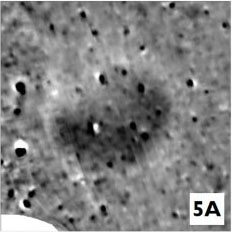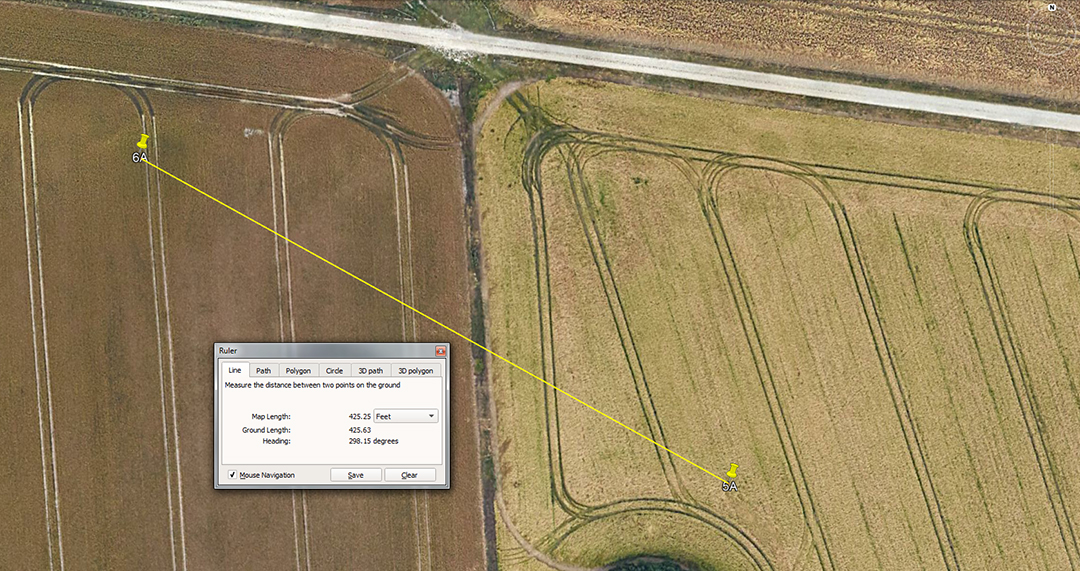The distance is 425.25-feet and the return angle is 118.125-degrees.

A mathematical progression based upon 425.25-feet goes:

425.25, 850.5, 1275.75, 1701 ... 2551.5 ... 3402 ... 6804, etc.

This string is easily identified as intervals of 1/8th, 1/4th, 1/2 and the full duration of the 6804-day lunar nutation cycle. The interval of 2551.5-days is, of course the lunar period of 7.2-lunar years that is monitored alongside 2556.75-days (7 solar years of 365.25-days each) under the Sabbatical Calendar system.

The angle of 118.125 is in homage to 4 lunar months of 29.53125-days. On the Calendar of Coligny brass plaque found in France in the late 19th century, readings could be done over 1890 days. Of this the solar count was 1890 ÷ 63 = 30-days for a solar month or 1890 ÷ 64 = 29.53125-days for a lunar month. Note also, the Great Pyramid length is 756-feet or 189-feet X 4 and 756-feet = 25.6 X 29.53125-feet.

6A TO 7A

51 10 56.93 N 1 47 34.26 W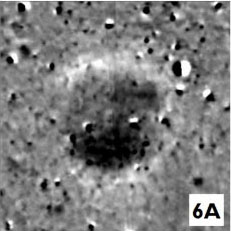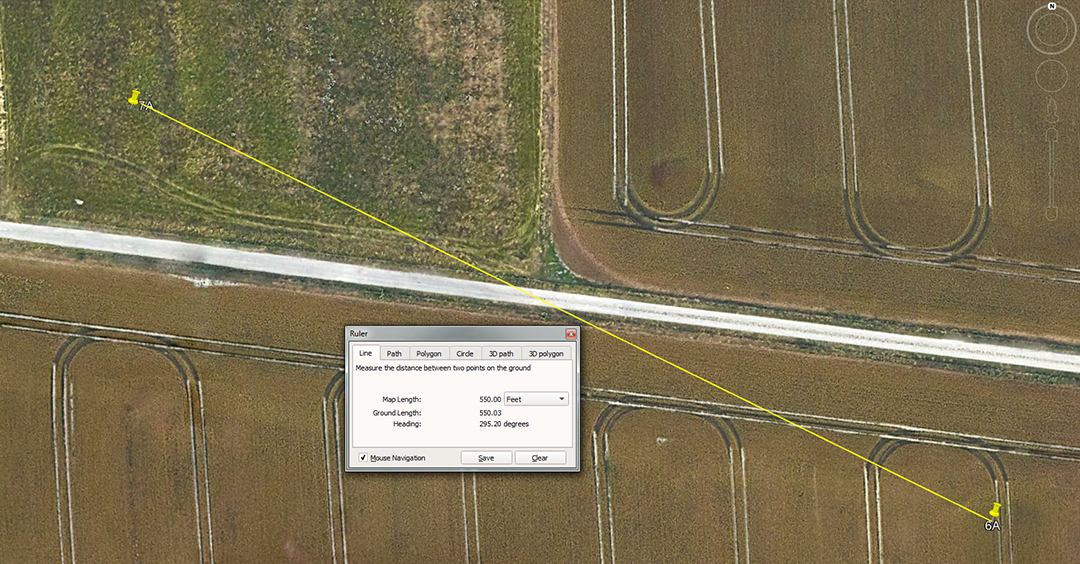The distance is 550-feet and the angle is 295.3125-degrees or a return angle also read as 115.2-degrees.

A mathematical progression based upon 550-feet goes:

550, 1100, 1650, 2200, 2750, 3300, 3850, 4400, 4950, 5500, 6050, 6600 ... 7700 ... 8800 ... 9900 ... 14850 ... 16500, etc.

The length of the Great Pyramid could be read as either 756-feet (literal) or 756.25-feet (symbolic ... 3-inches longer). At 756-feet 1-minute of equatorial arc for the Earth was 6048-feet (2 circumnavigations of the Great Pyramid or 12 X 12 X 12 X 12 X 1.2 miles of 5250-feet (24883.2-Greek miles ...130636800-feet).

However, if the pyramid was considered to be 3-inches longer (undoubtedly marked as such in the paving slabs at the base) one minute of equatorial arc was 6050-feet (24750 English-miles of 5280-feet ... 130680000-feet).

These were the two main navigational methods employed in antiquity, one based upon the numbers 6&7 combined (5250-feet mile) and the other based upon the "11" family of numbers (5280-feet mile).

In the above lineup, 5.5-feet was the ancient merchant navy fathom into the 20th century, 66-feet is the chain measurement, 660-feet is the furlong or furrowlong and 16500-feet is the ancient league (3.125-miles).

The angle is the very important value related to the lunar month. With a tiny variation, the return angle, 180-degrees opposed, would be read as 115.2-degrees. Under the 24883.2-mile assignment the sum of 115.2-miles would be 1/216th of the equatorial circumference.

The merits of 560-feet would also have featured in the tutorials related to the distances between these 2 shafts, as well as 562.5-feet.

A mathematical progression based upon 560 goes:

560, 1120, 1680, 2240, 2800, 3360, 3920, 4480, 5040, 5600 ... 6720 ... 10080 ... 14000 ... 15120 ... 30240, etc.

Again, we generate values used in traditional weights standard, as well as navigation. The Aubrey circle at Stonehenge was made up of 56 post markers and that value is very convenient for calculating lunar periods within the 6804-day lunar nutation cycle. Therefore: 6804 ÷ 56 = 121.5-days.

The ancient Irish mile was 2240-yards of 3-feet each or 6720-feet.

A progression based upon 562.5-feet of distance between shafts goes:

562.5, 1125, 1687.5, 2250 ... 4500 ... 6187.5 ... 9000 ... 11812.5 ...18000 ... 19687.5 ... 24750 ... 27000 ... 35437.5 ... 36000.

It can be seen that this string contains very important numbers in the 360-degree angle system, as well as lunar codes.

In the above lineup is the value 6187.5. The base length per side of the Bent Pyramid of Egypt was 618.75-feet, for a full perimeter value of 2475-feet. This coded the 24750-mile equatorial circumference under the navigational system that used an English mile of 5280'. The official length measurement is 188.6 metres for the Bent Pyramid's base, which translates to 618.75-feet.

Also, the value 19687.5 occurs in the above string. Under the ancient gold standard adopted by the Egyptians 196.875-grains was the weight of 1 Beqa of gold. The value of the Roman Uncia is 2 Egyptian Beqa (a gold weight standard value used in the Nubian goldfields and adopted by several civilisations). The Greek assayers also used the Beqa, which they called a Tridrachm @ 196.875 grains. The Roman Beqa, of equal grain weight to the Tridrachm or Egyptian Beqa, was called a Semuncia.

FROM 7A TO 8A

51 10 59.2 N 1 47 42.16 W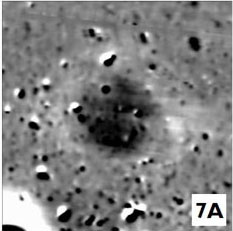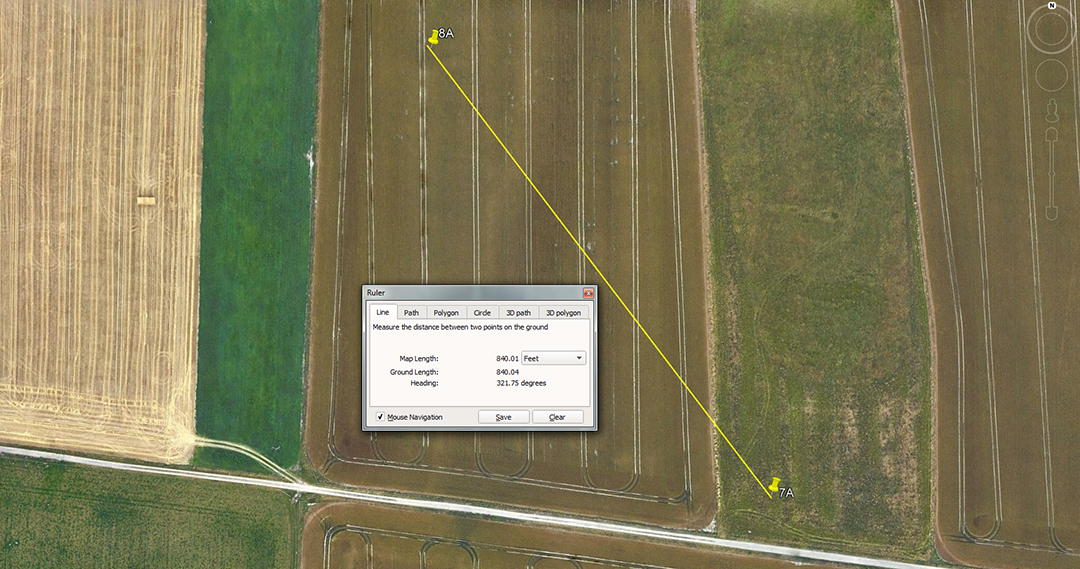The distance is 840-feet and the angle is 141.75-degrees return.

A mathematical progression based on 840 goes:

840, 1680, 2520, 3360, 4200, 5040, 5880, 6720, 7560, 8400, 9240, 10080 ... 12600 ... 15120 ... 30240, etc.

One can readily see Great Pyramid and associated navigational codes in this lineup.

The return angle for this shaft-to-shaft leg is 141.75-degrees and a mathematical progression based on this goes:

141.75, 283.5, 425.25, 567, 708.75, 850.5... 1134 ... 1417.5 ... 1701 ... 2268 ... 2551.5 ... 2835 ... 3402 ... 3543.75 ... 4536 ... 6804, etc.

These values are substantially lunar and relate to the base dimensions of the Khafre Pyramid of Egypt (pyramid of the moon). A circumnavigation of Khafre @ 708.75-feet per side = 2835-feet for all 4 sides. Also in the lineup are 1/8th, 1/4th, 1/2 and the full duration of the 6804-day lunar nutation cycle. The sum of 2268-feet would be 3 side values of the Great Pyramid @ 756-feet per side and the top altar floor of the pyramid reaches 453.6-feet of vertical height ... 264 Royal Cubits or 4.5 seconds of equatorial arc.

the merits of 850.5-feet would also have featured in the tutorial about the distance between the two shaft positions.

8A TO 9A

51 11 05.62 N 1 47 50.23 W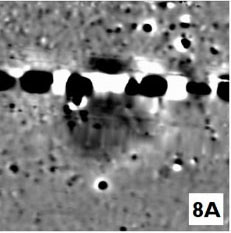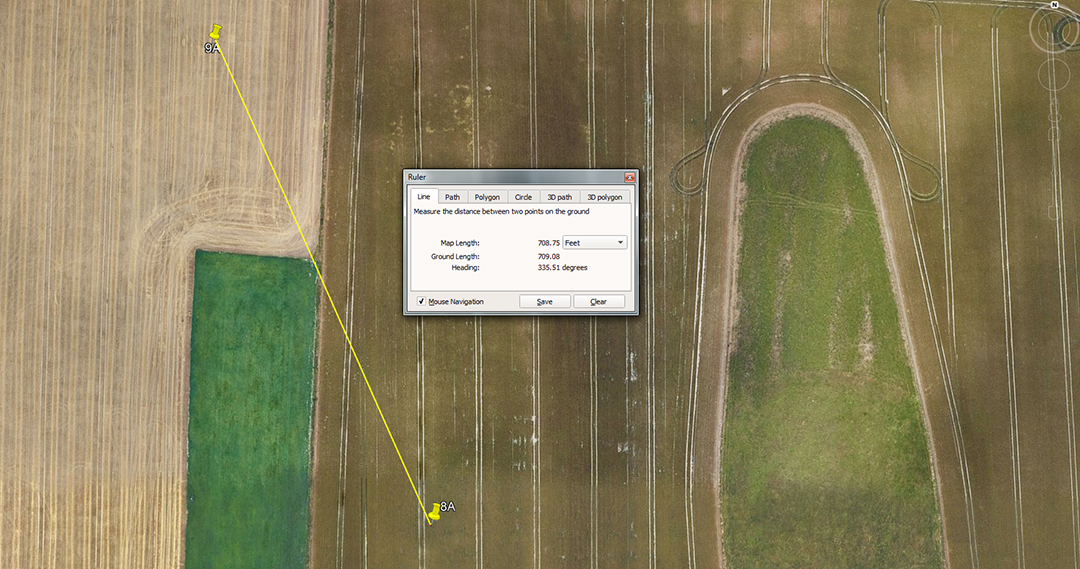The distance is 708.75-feet and the return angle is 155.52-degrees.

The distance is in homage to the length of the Khafre Pyramid (708.75 feet) which is, in days, 2 lunar years.

The angle is in homage to the equatorial circumference of the Earth, where a distance of 155.52-miles would be 1/160th. Therefore 24883.2 ÷ 155.52 = 160 or 2.25-degrees of arc.

The distance of 704-feet would also have featured:

704, 1408, 2112, 2816, 3520, 4224, 4928, 5632, 6336 ... 10560 ...52800, etc.

There would be 7.5 increments of 704-feet in a mile of 5280-feet and the value 63360-feet would be 12-miles.

Also, if the 130680000-feet equatorial circumference (24750-miles of 5280-feet) was converted into Egyptian Royal cubits of 20.625-inches, that value (76032000) ÷ 6336 = 12000. Alternatively, if divided by 5280 the total would be 14400. This shows the conversion interplay in readings of either feet or cubits of various kinds in the ratio-related ancient metrology systems.

9A TO THE EASTERN CURSUS POND.

51 11 11.93 N 1 47 54.94 W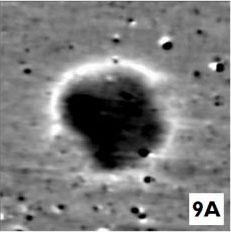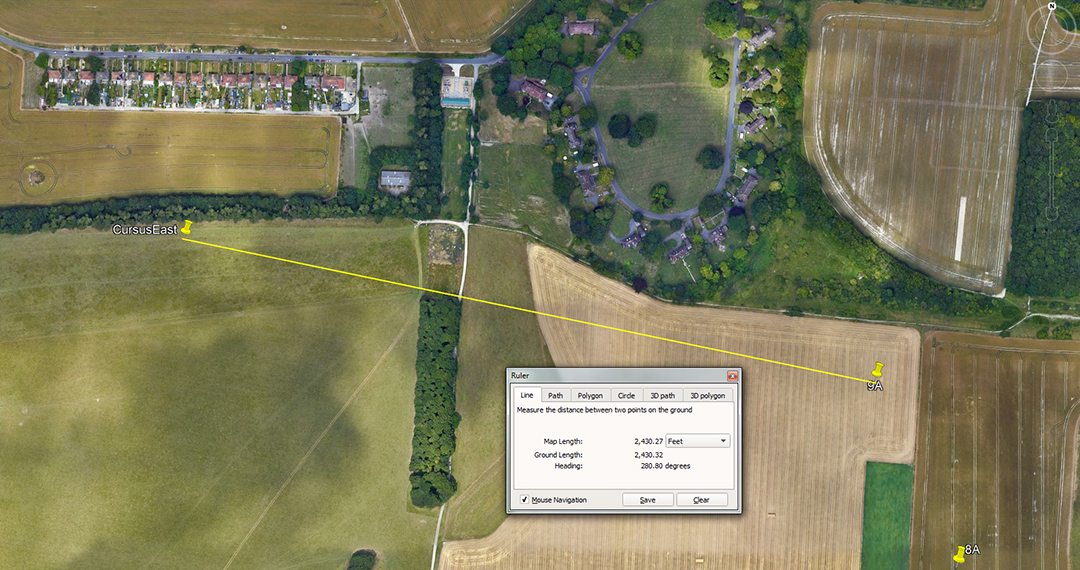The distance is 2430-feet and the return angle is 100.8-degrees.

A mathematical progression based upon 243 goes:

243, 486, 729, 972, 1215, 1458, 1701, 1944, 2187, 2430, 2673, 2916 ... 3402 ... 3645 ... 3888 ... 5832 ... 6804 ... 7776 ... 8505 ... 11664 ... 13608 ... 15552 ... 25515. etc.

This is a highly important string, loaded with lunar, navigational or calendar codes.

The Druidic brass plaque called the Calendar of Coligny measures 4.86-feet of length and this equates to a Roman Pace of 58.32-inches (5 Roman feet of 11.664-inches) The Roman mile, adopted from much earlier nations, was 5000 Roman feet or 4860-feet of 12-inches each. The value 7.29-inches was 1/8th of a Roman pace and this is the length of the Bush Barrow Lozenge artefact exhumed from a tumulus mound within a stone's throw of Stonehenge. The grave in which the lozenge was found dates to about 2000 BC. The width of the lozenge is based upon the PHI reciprocal and is 6.1804-inches, the dynamic purpose of which will be explained as we proceed.

The Roman foot was found to be 11.664 inches by John Greaves, professor of geometry, who in 1639 went to Rome specifically to ascertain the length of an ancient Roman foot. Greaves located a monument of Roman architect, Stalius Asper and measured bas relief instruments used by him in the first century A. D. Greaves concluded, after careful investigation, that the Roman foot, 'contained 1944 such parts as the English foot contains 2000'. This means a Roman foot of 11.664 inches.

In the lineup of this string occurs, once again, the values for 1/4th (1701-days), 1/2 (3402-days), 3/4ths (5103-days) and the full duration (6804-days) of the lunar nutation cycle.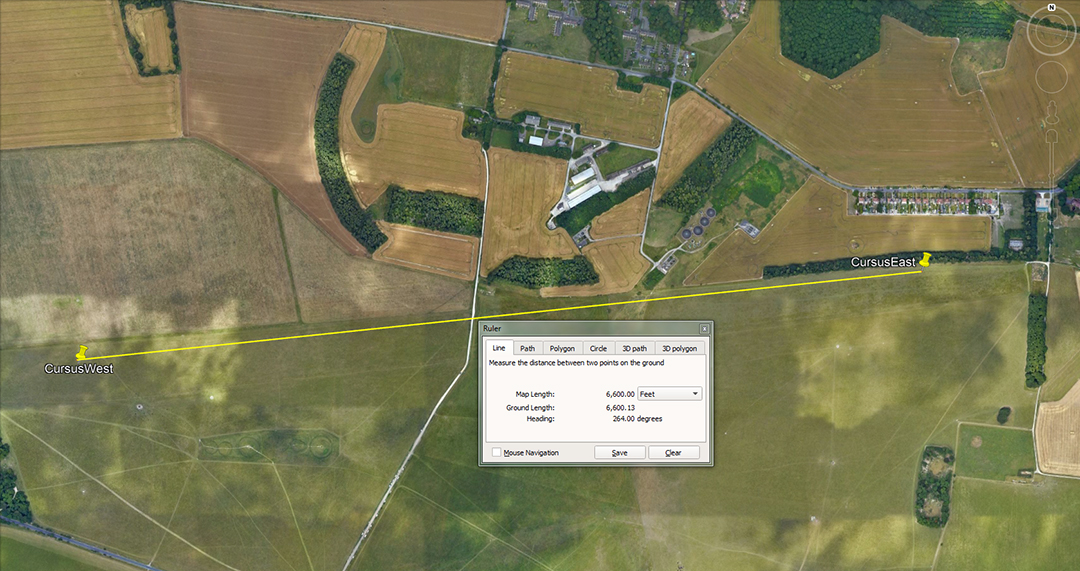While in the vicinity of the Greater Cursus, it's timely to identify the distance and angle coding between the east and west end pits. They sit 10 furlongs from each other (6600-feet or 1.25 miles of 5280-feet) at an angle (east to west) of 264-degrees.

9A TO ii

51 11 11.93 N 1 47 54.94 W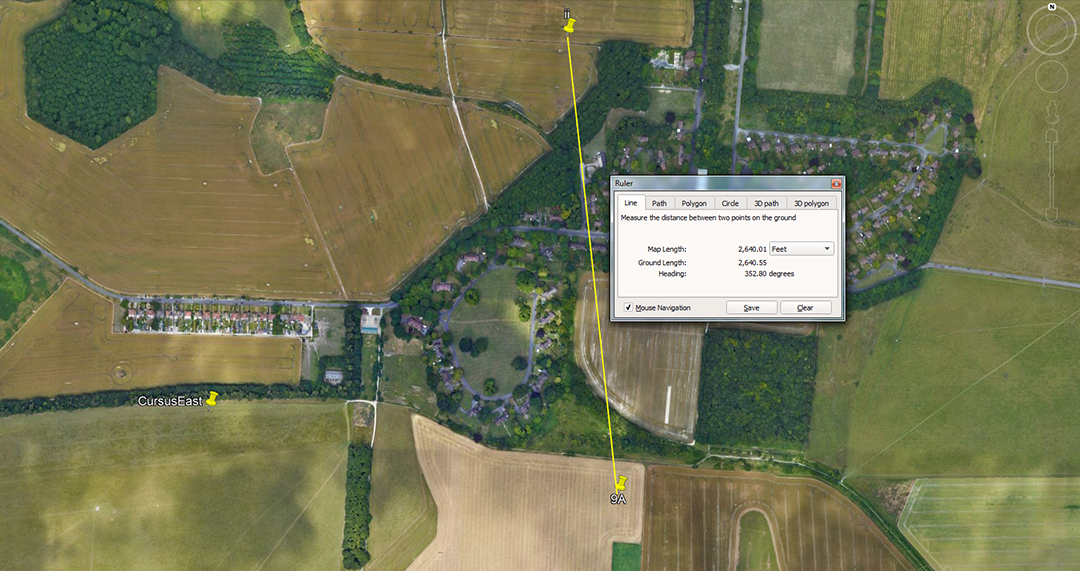The distance is 2240-feet and the return angle is 172.8-degrees.

The distance is reminiscent of the 2240 value that became the English ton weight of 2240 1bs, still used by the Americans, along with the 5280-feet mile. A mathematical progression based upon 224 goes:

224, 448, 672, 896, 1120, 1344, 1568, 1792, 2016, 2240 ... 2688 ... 2912 ... 3360 ... 3584 ... 4032 ... 5600 ... 6048 ... 6720, etc.

The sum of 112 lbs became the hundredweight of which there were 20 per ton. The value of 2016-feet is 1/3rd of 1-minute of equatorial arc; 4032-feet is 2/3rds and 6048 is 1-minute of arc under the Great Pyramid's literal assignment. In this string the value 6720-feet was the length of the ancient Irish mile.

The angle of 172.8-degrees provides a very important mathematical progression:

172.8, 345.6, 518.4, 691.2, 864, 1036.8, 1209.6, 1382.4, 1555.2, 1728, 1900.8, 2073.6 ... 2592 ... 3110.4 ... 3628.8 ... 4147.2 ... 4320 ... 4665.6 ... 5184 ... 6048 ... 12441.6 ... 24883.2.

Firstly, Petrie found a repetitive measurement within the paving slabs of the Great Pyramid of 1.728-feet, which equated to 20.736-inches, the largest of 3 Royal cubits that he identified. Remember, these cubit rules, in their overall lengths, were only for encoding the equatorial circumference of the Earth. One read the inch value as miles, then multiplied that value by 1200. Therefore 20.736-miles X 1200 = 24883.2.

Also, a cubic foot = 12 X 12 X 12 inches or 1728-inches cubed. This is reminiscent of 12 X 12 X 12 X 12 X 1.2-miles = 24883.2, a mnemonic method of remembering the size of the Earth.

Again, 51.84-degrees is the slope angle of the Great Pyramid's faces and the area the pyramid's base covers is 72 X 72 reeds of 10.5-feet each or 5184 square reeds. Also, 5184-years is 1/5th of the duration of the 25920-year Precession of the Equinoxes. Likewise, the distance from the centre of Stonehenge to the outer face of the Heel Stone is 259.2-feet and the circumference of the Sarsen circle is 345.6-feet (11.52-feet X 30).

When navigating at sea in leagues of 16500-feet (3.125-miles), the straight line distance converted to 51840-feet using PI @ 1728/550ths (3.141818182).

It's of note that the base of Silbury Hill is slightly oval to provide two 90-degrees opposed coded diameters. One of these is 525-feet in diameter and the other is 550-feet. The 525-feet diameter became 1 short Greek stadia and for converting this combination of "6&7" number families into a whole number circumference one uses PI @ 22/7ths = 1650-feet. Alternatively, if one wishes to turn 550-feet (11 family of numbers) into a whole number circumference one uses PI @ 1728/550ths = 1728. In other words, Silbury hill was considered to be 1650-feet (55-inches X 360) in circumference, based upon a diameter of 525-feet or 1728-feet (4.8-feet or 57.6-inches X 360) in circumference, based upon a diameter of 550-feet.

The base cross-measures of Silbury Hill near Avebury Henge was obviously used for tutorials about the versions of the PI ratio to use when navigating by the miles of 5250-feet or 5280-feet or their league equivalents.

FROM ii TO iii

51 11 37.76 N 1 48 00.31 W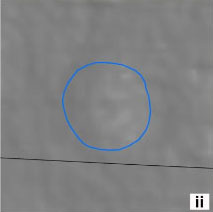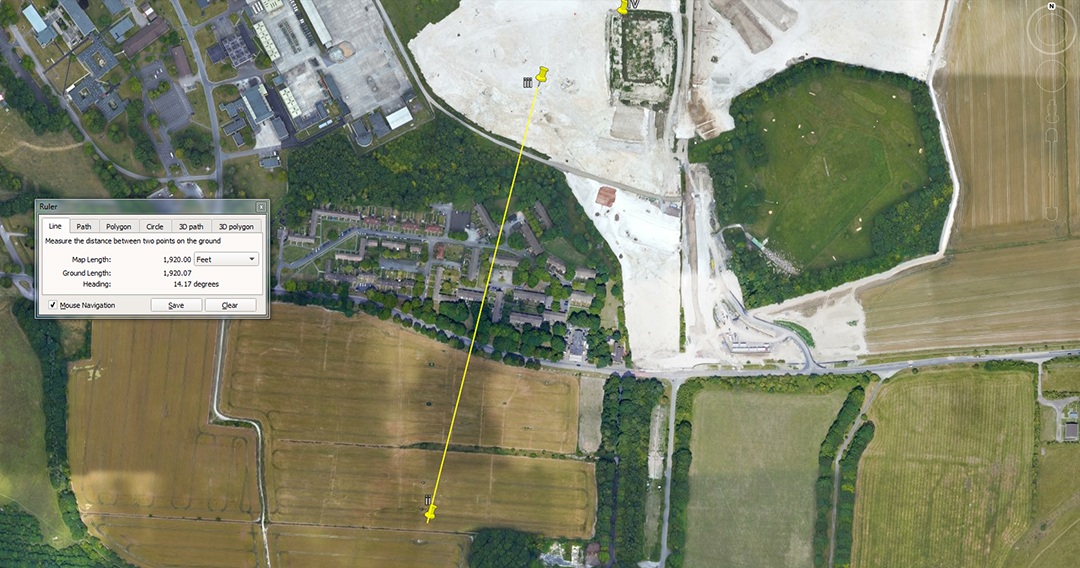The distance is1920-feet and the return angle is 14.175-degrees.

A mathematical progression based upon 192 goes:

192, 384, 576, 768, 960, 1152, 1344, 1536, 1728, 1920, 2112, 2304 ... 2268 ... 2880 ... 3456 ... 4032 ... 4608 ... 4800 ... 5184 ... 6912 ... 10560 ... 20736 ... 25920, etc.

As can be seen the values relate to navigation, precession of the equinoxes, and the equatorial circumference of the Earth. The value 384-feet best describes the overall width of Stonehenge in one cross measure that encloses the embankments. The protruding Avenue extends beyond that of course and the embankment circle is slightly oval.

With the Great Pyramid's navigational system configuring the Earth to be 24883.2-miles of 5250-feet in circumference, 1-degree of arc equates to 69.12 Greek miles.

A mathematical progression based upon 141.75 goes:

141.75, 283.5, 567, 708.75, 850.5 ... 1134... 1701 ... 2268 ... 2551.5 ... 2835 ... 3402 ... 3543.75 ... 4536 ... 5103 ... 6804, etc.

Again, we have a lineup of strong lunar numbers related to the 6804-day lunar nutation cycle. Also, the value 2835 is the distance around the base of the Khafre Pyramid and 2835-days would be 8-lunar years of 354.375-days or 96 lunar months of 29.53125-days (29 & 17/32nds).

iii TO IV

51 11 56.18 N 1 47 52.85 W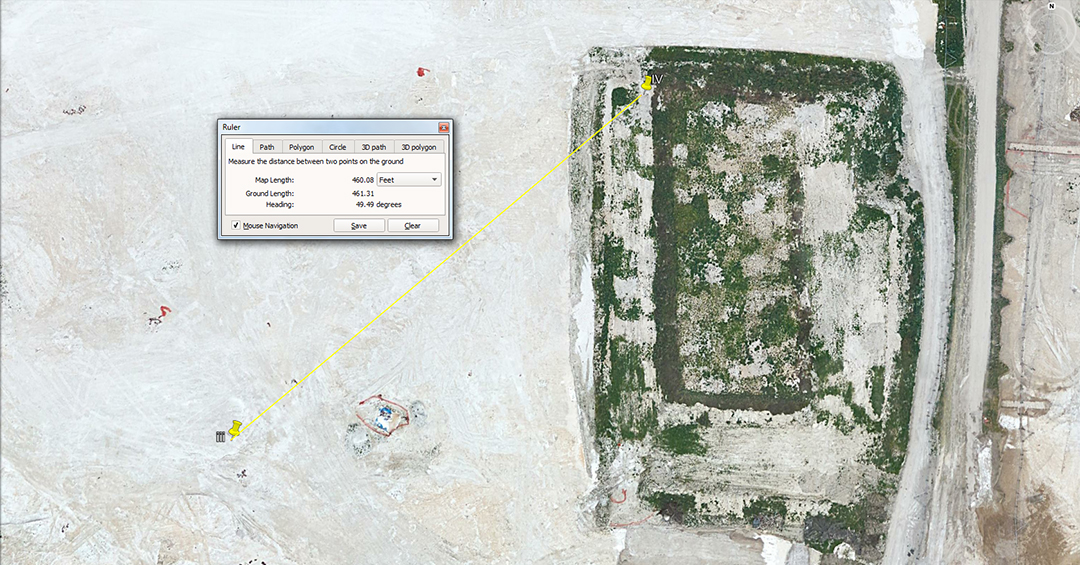The distance code is 460.8-feet and the angle code is 49.5-degrees.

A mathematical progression based upon 460.8 goes:

460.8, 921.6, 1382.4 ... 2304 ... 4147.2 ... 4608 ... 6912 ... 9216 ... 10137.6 ... 11520 ... 12441.6 ... 20736 ... 24883.2.

Again, we get a solid lineup of navigational codes based upon an Earth of 24883.2-miles of equatorial circumference.

Navigators at sea had to have easy-to-calculate navigational systems and the Great Pyramid coded two such systems. These were:

1. 130636800-feet of equatorial circumference, with 1-second of arc being 100.8-feet, 1-minute of arc being 6048-feet, and 1-degree of arc being 362880-feet. The system used 24883.2-miles of 5250-feet.

2. 130680000-feet of equatorial circumference, with 1-second of arc being 100.833333-feet, 1-minute of arc being 6050-feet and 1 degree of arc being 363000-feet. This system used 24750-miles of 5280-feet.

3. 131383296-feet of equatorial circumference, with 1-second of arc being 101.376-feet, 1-minute of arc being 6082.56-feet and 1-degree of arc being 364953.6-feet. This system used 24883.2-miles of 5280-feet.

Navigation by the first two systems was reasonably lacking in complexity, but there was a shortfall of 160.18-miles and 152-miles respectively in the two readings of the Earth's true equatorial circumference. This is because the scientists needed to work with numbers that could adequately describe a full circumference, but break it down fluidly to as little as 1-inch or fractions thereof with ease. They needed highly factorable numbers and would settle for minor error in the overall reading to achieve this.

However, they still wished to encode the full and true circumference and settled for 24883.2-miles of 5280-feet, which is only 18.8-miles short of the 24902-mile equatorial circumference figure that we use today.

The angle code is 49.5-degrees and a mathematical progression based upon this goes:

49.5, 99, 148.5, 198, 247.5, 297, 346.5, 396, 445.5, 495 ... 594 ... 792 ... 891 ... 990 ... 1188 ... 1584 ... 1782 ... 1980 ... 2376 ... 2475 ... 2970 ... 3564 ... 5940 ... 7920 ... 24750.

This string accentuates navigation by the ancient Scottish mile of 5940-feet, which was 1-furlong (660-feet) longer than the English mile. It is very evident that measurement increments of 2.475-inches or 2.475-feet were used on Megalithic-Nneolithic sites. At Stonehenge the "Y"-Holes Circle has a diameter of 178.2-feet, which is 864 increments of 2.475-inches or 72 increments of 2.475-feet (29.7-inches). Note, the half value of 5940 is 2970.

IV TO 10D

51 11 59.13 N 1 47 47.33 W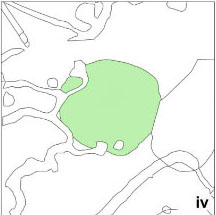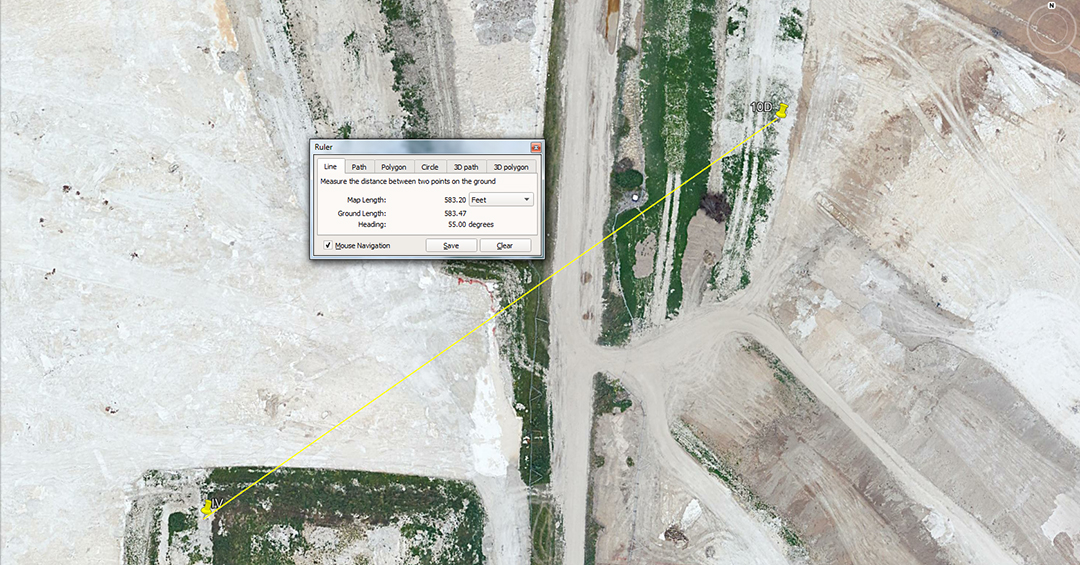The distance is 583.2-feet and the angle is 55-degrees. The merits of 594-feet and 590.625-feet would also have been taught.

The distance of 583.2-feet = 120 Roman Paces or 600 Roman feet ... marked into the English landscape over 2500-years before there was a Roman civilisation. A mathematical progression, based on 583.2 goes:

583.2, 1166.4, 1749.6, 2332.8, 2916, 3499.2, 4082.4, 4665.6 ... 5832 ... 8748 ... 9331.2 ... 11664 ... 14580 ... 46656 ... etc.

The Romans navigated by a different system, using a mile of 5000 Roman feet, based upon a foot read as 11.664-inches. Under a tradition that survived into mediaeval times in Europe, 75 Roman miles represented 1-degree of arc for the world.

The ancient Egyptian volume for a Theban was 11664 cubic inches and their Honnu was 116.64 cubic inches.

It's very apparent from a trades perspective that the foot of 11.664-inches was only for lunar readings or calculating precise, standard cubic inch volumes for capacity vessels (liquid or dry goods). For armies marching overland, the more reasonable foot reading would have conveniently been 11.66666-inches, meaning that every 5400 Roman feet length converted to 1 Greek mile of 5250-feet. This would also mean a Roman pace of 58.33333-inches.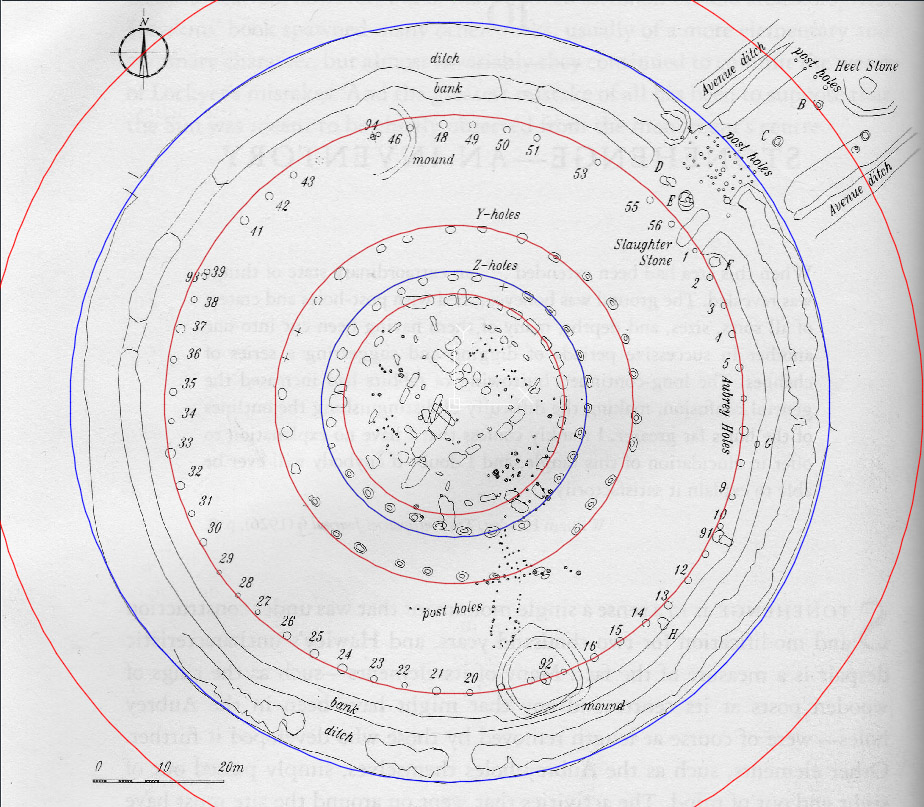The outer red circle sits a coded radius distance of 233.28-feet from the centre of Stonehenge and its outer rim is marked by stone B on the Avenue. This means a marked diameter for a circle of 466.56-feet or 96 Roman paces (480 Roman feet of 11.664-inches each).

Each of the red circles shown is in a close PHI (1.6180339 to 1) reducing relationship to its outer predecessor. Therefore, The outermost circle (466.56-feet) ÷ PHI = 288.35- feet (the Aubrey Circle of posts is coded to be 288-feet in diameter) ÷ PHI = 178.21 (the "Y" Holes circle is coded to be 178.2-feet in diameter) ÷ PHI = 110.1 (falling centrally on the lintels of the Sarsen Circle).

The "Z"-Holes Circle is not included in the PHI reduction and has a diameter of 132-feet or 1/40th of a mile of 5280-feet.

In the above mathematical progression about the merits of 583.2-feet, its of interest to note that the ancient Sumerian/ Babylonian Talent weight was 933120-grains.

10D TO 11D

51 12 02.39 N 1 47 39.81 W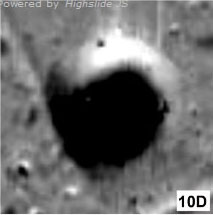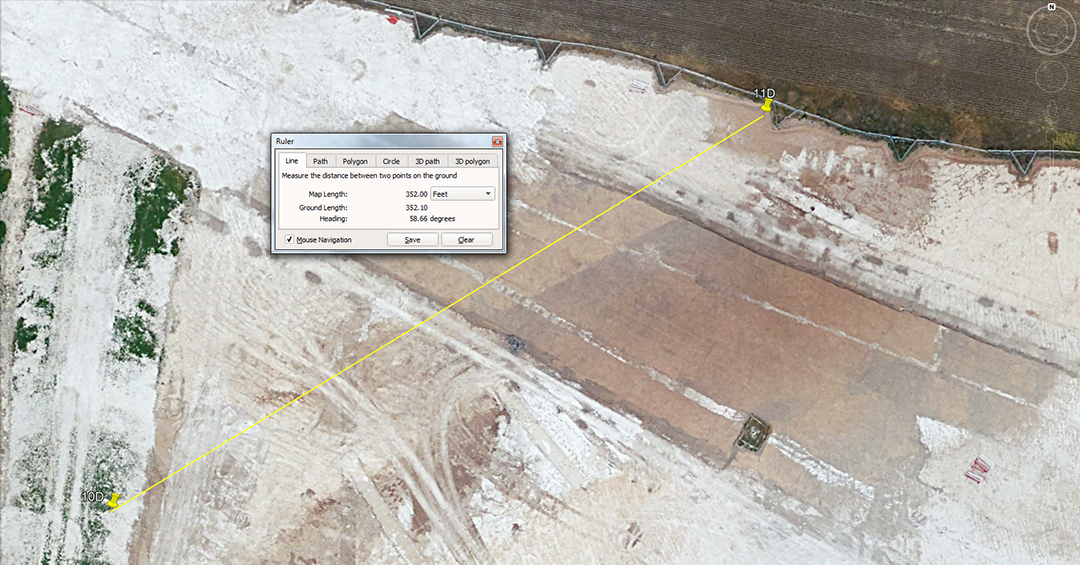The distance is 352-feet and the angle is 58.666-degrees. The merits of 354.375-feet also featured, as well as 356.4. The angle of 59.0625-degrees would also have been taught at this juncture.

A mathematical progression based upon 352 goes:

352, 704, 1056, 1408, 1760, 2112, 2464, 2816, 3168, 3520, 3872, 4224. 4576, 4928, 5280, 5632, 5984, 6336 ... 26400 ... 52800, etc.

The string relates to the 5280-feet mile of which 352-feet would be 1/15th, 1760 would be 1/3rd and 6336-feet would be 1.2-miles.

The angle of 58.6666-degrees provides the following similar progression:

58.666666, 117.33333, 176, 214.66666, 293.33333, 352 ... 528 ... 1760 ... 5280, etc.

The sum of 58.66666-feet would be 1/90th of an English mile of 5280-feet, in much the same way as 58.33333 would be 1/90th of a Greek mile of 5250-feet.

Under the Earth equatorial assignment of 24883.2-miles of 5280-feet (131383296-feet) 1-second of arc is 101.376-feet or 58.66666 Egyptian Royal Cubits of 20.376-inches.

A mathematical progression based upon 356.4 goes:

356.4, 712.8, 1069.2, 1425.6, 1782, 2138.4, 2494.8, 2851.2, 3207.6, 3564 ... 5346 ... 7128 ... 8910 ... 130680000.

Another mile used anciently was the Scottish mile (5940-feet), which was 660-feet (1-furlong) longer than the English mile of 5280-feet. These miles were used to describe the equatorial circumference as either 22000 Scottish miles of 5940-feet or 24750 English miles of 5280-feet. There were, quite obviously, increments of 2.475-inches or 2.475-feet (297-inches) used on the many British sites for Scottish mile related calculations.

The sum of 3564-feet would be 17280 increments of 2.475-inches in 3564-feet or 1440 increments of 2.475-feet (297-inches). In the full equatorial circumference under this navigational assignment 130680000-feet there would be 52800000 increments of 2.475-feet.

It seems obligatory that the tutorial would have elaborated on the close proximity distance of 354.375-feet, as well as an angle of 59.0625-degrees, both strong lunar codes.

11D TO 12D

51 12 04.19 N 1 47 35.09 W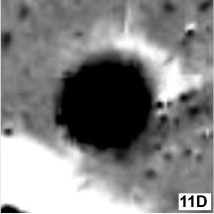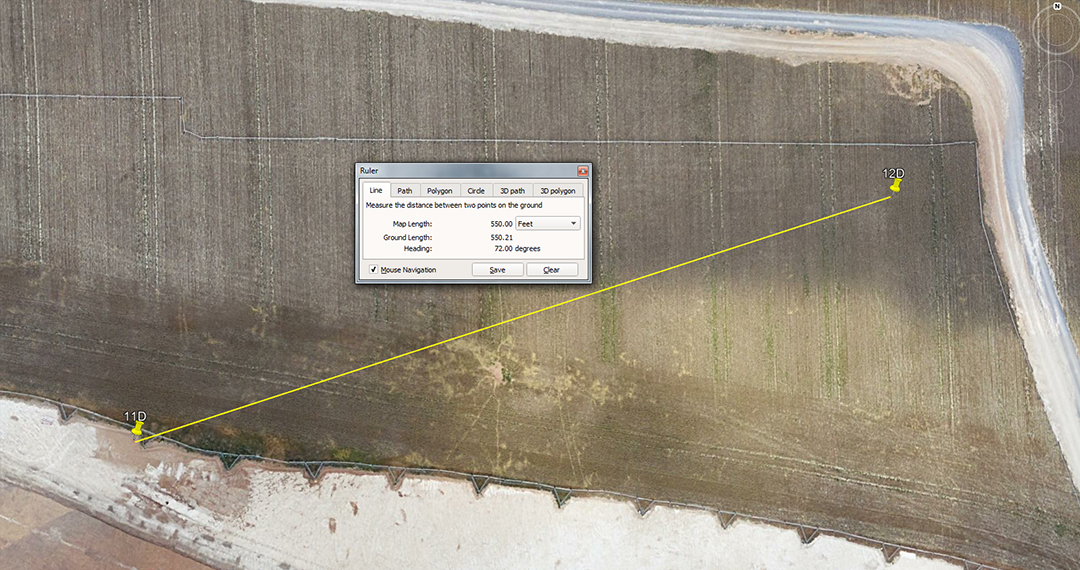The distance is 350-feet and the angle is 72-degrees (return angle 252-degrees). The distance would also have been read as 354.375-feet, as well as 352-feet.

A progression based upon 35 goes:

35, 70, 105, 140, 175, 210, 245, 280, 315, 350, 385, 420, 455, 490, 525, 560, 595, 630, 665, 700, 735, 770 ... 840 ... 875 ... 945 ... 1120 ... 1260 ... 5250 ... 6300.

The above lineup identifies measurement increments that became the basis for late era navigation by Greek mariners or overland surveying by their landlocked counterparts.

The sum of 10.5-feet (126-inches) was the Greek parallel to the Hebrew reed; 315-feet was 1/2 of a long stadia and was also used as a form of PI @ 3.15; The length of 525-feet was the short stadia and 630 feet was the long stadia and the length marked into the running track at Olympus for the sprint event; The 5250-feet length became the Greek mile, although it was also used by more ancient predecessors for thousands of years before there was a Greek civilisation.

The degree angle read as 252-degrees return also provides a mathematical progression that accentuates navigational numbers found within the Great Pyramid's dimensions:

252, 504, 756, 1008, 1260, 1512, 1764, 2016, 2268, 2520, 2772, 3024, 3276, 3528, 3780, 4032 ... 4536 ... 6048 ... 6300 ... 6804 ... 9072 ... 13608 ... 18900.

The ancient Assyrian cubit was two Greek feet or 25.2-inches; 756 feet is the design length of the Great Pyramid or 1/8th of 1-second of equatorial distance around the Earth; 100.8-feet is 1-second of equatorial arc; 2016, 4032 and 6048-feet are 1/3rd, 2/3rds and a full minute of equatorial arc, respectively; 453.6-feet is the vertical height of the truncated Great Pyramid or 4.5 seconds of arc, as well as 264 Royal cubits of 20.618182-inches.

The value 6804, of course, gives us the number used to describe the duration, in days, of the lunar nutation cycle, essential knowledge for tracing the daily progress of the moon under the lunisolar Sabbatical calendar system.

12D TO 13D

51 12 05.85 N 1 47 26.85 W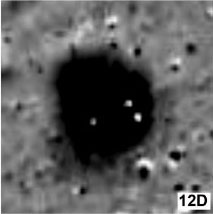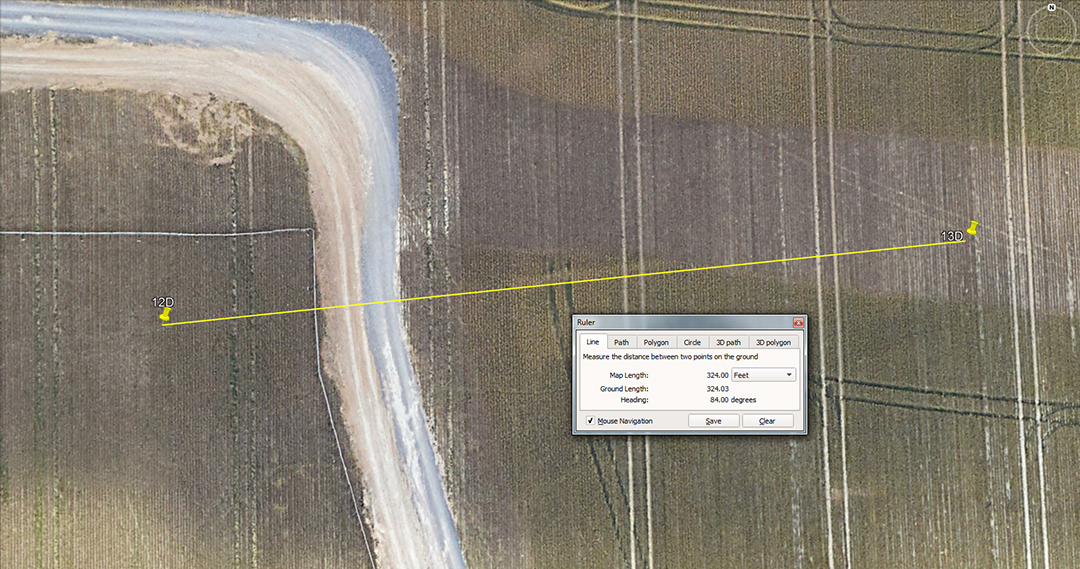The distance is 324-feet and the angle is 84-degrees. The merits of 320' would also have featured' as well as 264-degrees return.

A progression based upon 324 goes:

324, 648, 972, 1296, 1620, 1944, 2268, 2592, 2916, 3440, 3564, 3888 ... 4536 ... 4860 ... 5184 ... 5832 ... 6804 ... 7776 ... 9072 ... 10368 ... 11340 ... 11664 ... 12960 ... 25920.

This string provides a raft of essential numbers for calculating periods within the 25920-year duration of the Precession of the Equinoxes, as well as navigational codes as found within the dimensions and slope angle of the Great Pyramid. Also, 10368-feet is the coded distance from the centre of Durrington Walls Henge to the centre of Stonehenge. Alternatively, the sum of 1036.8 miles per hour is the speed at which the 24883.2-mile around Earth orb spins in 24-hours. The lineup provides values used for the Roman foot or pace (11.664 & 58.32-inches respectively), as well as the value 162, which in the form of 1.62 worked as a rounded expression of PHI (1.6180339 to 1) in much the same way as the Greek half stadia (315-feet) was used as a rounded form of PI @ 3.15.

The angle value of 84-degrees sets up the following progression:

84, 168, 252, 336, 420, 504, 588, 672, 756, 840, 924, 1008 ... 1260 ... 1344 ... 1512 ... 2016 ... 3024 ... 3780 ... 4032 ... 4536 ... 6048 ... 6720, etc.

Again, very recognisable numbers that relate to the Great Pyramid's literal system of navigation, including the ancient Irish mile of 6720-feet.

Continue